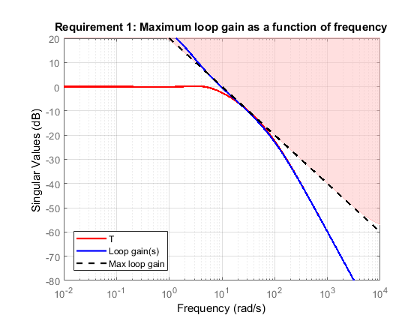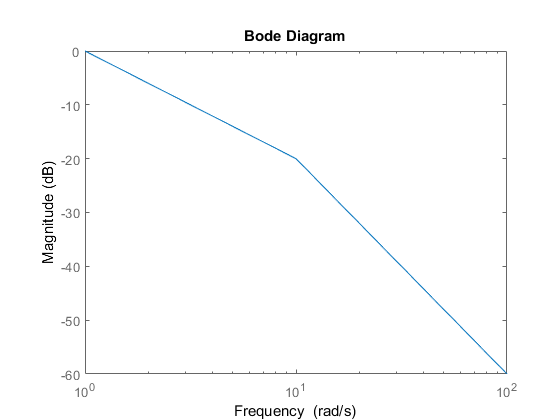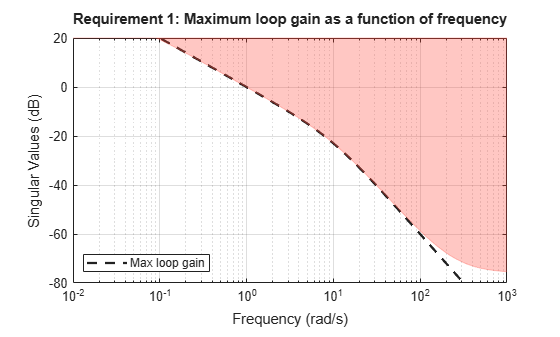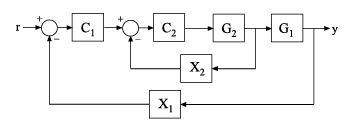Documentation

# TuningGoal.MaxLoopGain class

Package: TuningGoal

Maximum loop gain constraint for control system tuning

## Description

Use `TuningGoal.MaxLoopGain` to enforce a maximum loop gain and desired roll-off in a particular frequency band. Use this tuning goal with control system tuning commands such as `systune` or `looptune`.

This tuning goal imposes a maximum gain on the open-loop frequency response (L) at a specified location in your control system. You specify the maximum open-loop gain as a function of frequency (a maximum gain profile). For MIMO feedback loops, the specified gain profile is interpreted as an upper bound on the largest singular value of L.

When you tune a control system, the maximum gain profile is converted to a maximum gain constraint on the complementary sensitivity function, T) = L/(I + L).

The following figure shows a typical specified maximum gain profile (dashed line) and a resulting tuned loop gain, L (blue line). The shaded region represents gain profile values that are forbidden by this tuning goal. The figure shows that when L is much smaller than 1, imposing a maximum gain on T is a good proxy for a maximum open-loop gain.`TuningGoal.MaxLoopGain` and `TuningGoal.MinLoopGain` specify only high-gain or low-gain constraints in certain frequency bands. When you use these tuning goals, `systune` and `looptune` determine the best loop shape near crossover. When the loop shape near crossover is simple or well understood (such as integral action), you can use `TuningGoal.LoopShape` to specify that target loop shape.

## Construction

`Req = TuningGoal.MaxLoopGain(location,loopgain)` creates a tuning goal for limiting the gain of a SISO or MIMO feedback loop. The tuning goal limits the open-loop frequency response measured at the specified locations to the maximum gain profile specified by `loopgain`. You can specify the maximum gain profile as a smooth transfer function or sketch a piecewise error profile using an `frd` model or the `makeweight` command. Only gain values smaller than 1 are enforced.

`Req = TuningGoal.MaxLoopGain(location,fmax,gmax)` specifies a maximum gain profile of the form `loopgain = K/s` (integral action). The software chooses `K` such that the gain value is `gmax` at the specified frequency, `fmax`.

### Input Arguments

 `location` Location at which the maximum open-loop gain is constrained, specified as a character vector or cell array of character vectors that identify one or more locations in the control system to tune. What loop-opening locations are available depends on what kind of system you are tuning:If you are tuning a Simulink® model of a control system, you can use any linear analysis point marked in the model, or any linear analysis point in an `slTuner` interface associated with the Simulink model. Use `addPoint` to add analysis points to the `slTuner` interface. For example, if the `slTuner` interface contains an analysis point `u`, you can use `'u'` to refer to that point when creating tuning goals. Use `getPoints` to get the list of analysis points available in an `slTuner` interface to your model.If you are tuning a generalized state-space (`genss`) model of a control system, you can use any `AnalysisPoint` location in the control system model. For example, the following code creates a PI loop with an analysis point at the plant input `'u'`. ```AP = AnalysisPoint('u'); G = tf(1,[1 2]); C = tunablePID('C','pi'); T = feedback(G*AP*C,1); ```When creating tuning goals, you can use `'u'` to refer to the analysis point at the plant input. Use `getPoints` to get the list of analysis points available in a `genss` model. If `location` is a cell array of loop-opening locations, then the maximum gain requirement applies to the resulting MIMO loop. `loopgain` Maximum open-loop gain as a function of frequency. You can specify `loopgain` as a smooth SISO transfer function (`tf`, `zpk`, or `ss` model). Alternatively, you can sketch a piecewise gain profile using a `frd` model or the `makeweight` command. For example, the following `frd` model specifies a maximum gain of 1 (0 dB) at 1 rad/s, rolling off at a rate of –20 dB/dec up to 10 rad/s, and a rate of –40 dB/dec at higher frequencies. ```loopgain = frd([1 1e-1 1e-3],[1 10 100]); bodemag(loopgain) ```When you use an `frd` model to specify `loopgain`, the software automatically maps your specified gain profile to a `zpk` model. The magnitude of this model approximates the desired gain profile. Use `viewGoal(Req)` to plot the magnitude of that `zpk` model. Only gain values smaller than 1 are enforced. For multi-input, multi-output (MIMO) feedback loops, the gain profile is interpreted as a minimum roll-off requirement, which is an upper bound on the largest singular value of `L`. For more information about singular values, see `sigma`. If you are tuning in discrete time (that is, using a `genss` model or `slTuner` interface with nonzero `Ts`), you can specify `loopgain` as a discrete-time model with the same `Ts`. If you specify `loopgain` in continuous time, the tuning software discretizes it. Specifying the loop gain in discrete time gives you more control over the loop gain near the Nyquist frequency. `fmax` Frequency of maximum gain `gmax`, specified as a scalar value in rad/s. Use this argument to specify a maximum gain profile of the form ```loopgain = K/s``` (integral action). The software chooses `K` such that the gain value is `gmax` at the specified frequency, `fmax`. `gmax` Value of maximum gain occurring at `fmax`, specified as a scalar absolute value. Use this argument to specify a maximum gain profile of the form ```loopgain = K/s``` (integral action). The software chooses `K` such that the gain value is `gmax` at the specified frequency, `fmax`.

## Properties

 `MaxGain` Maximum open-loop gain as a function of frequency, specified as a SISO `zpk` model. The software automatically maps the input argument `loopgain` onto a `zpk` model. The magnitude of this `zpk` model approximates the desired gain profile. Alternatively, if you use the `fmax` and `gmax` arguments to specify the gain profile, this property is set to `K/s`. The software chooses `K` such that the gain value is `gmax` at the specified frequency, `fmax`. Use `viewGoal(Req)` to plot the magnitude of the open-loop maximum gain profile. `Focus` Frequency band in which tuning goal is enforced, specified as a row vector of the form `[min,max]`. Set the `Focus` property to limit enforcement of the tuning goal to a particular frequency band. Express this value in the frequency units of the control system model you are tuning (rad/`TimeUnit`). For example, suppose `Req` is a tuning goal that you want to apply only between 1 and 100 rad/s. To restrict the tuning goal to this band, use the following command:`Req.Focus = [1,100];` Default: `[0,Inf]` for continuous time; `[0,pi/Ts]` for discrete time, where `Ts` is the model sample time. `Stabilize` Stability requirement on closed-loop dynamics, specified as 1 (`true`) or 0 (`false`). When `Stabilize` is `true`, this requirement stabilizes the specified feedback loop, as well as imposing gain or loop-shape requirements. Set `Stabilize` to `false` if stability for the specified loop is not required or cannot be achieved. Default: 1 (`true`) `LoopScaling` Toggle for automatically scaling loop signals, specified as `'on'` or `'off'`. In multi-loop or MIMO control systems, the feedback channels are automatically rescaled to equalize the off-diagonal terms in the open-loop transfer function (loop interaction terms). Set `LoopScaling` to `'off'` to disable such scaling and shape the unscaled open-loop response. Default: `'on'` `Location` Location at which minimum loop gain is constrained, specified as a cell array of character vectors that identify one or more analysis points in the control system to tune. For example, if ```Location = {'u'}```, the tuning goal evaluates the open-loop response measured at an analysis point `'u'`. If ```Location = {'u1','u2'}```, the tuning goal evaluates the MIMO open-loop response measured at analysis points `'u1'` and `'u2'`. The value of the `Location` property is set by the `location` input argument when you create the tuning goal. `Models` Models to which the tuning goal applies, specified as a vector of indices. Use the `Models` property when tuning an array of control system models with `systune`, to enforce a tuning goal for a subset of models in the array. For example, suppose you want to apply the tuning goal, `Req`, to the second, third, and fourth models in a model array passed to `systune`. To restrict enforcement of the tuning goal, use the following command: `Req.Models = 2:4;` When `Models = NaN`, the tuning goal applies to all models. Default: `NaN` `Openings` Feedback loops to open when evaluating the tuning goal, specified as a cell array of character vectors that identify loop-opening locations. The tuning goal is evaluated against the open-loop configuration created by opening feedback loops at the locations you identify. If you are using the tuning goal to tune a Simulink model of a control system, then `Openings` can include any linear analysis point marked in the model, or any linear analysis point in an `slTuner` interface associated with the Simulink model. Use `addPoint` to add analysis points and loop openings to the `slTuner` interface. Use `getPoints` to get the list of analysis points available in an `slTuner` interface to your model. If you are using the tuning goal to tune a generalized state-space (`genss`) model of a control system, then `Openings` can include any `AnalysisPoint` location in the control system model. Use `getPoints` to get the list of analysis points available in the `genss` model. For example, if `Openings = {'u1','u2'}`, then the tuning goal is evaluated with loops open at analysis points `u1` and `u2`. Default: `{}` `Name` Name of the tuning goal, specified as a character vector. For example, if `Req` is a tuning goal: `Req.Name = 'LoopReq';` Default: `[]`

## Examples

collapse all

Create a tuning goal that limits the maximum open-loop gain of a feedback loop to a specified profile.

Suppose that you are tuning a control system that has a loop-opening location identified by `PILoop`. Limit the open-loop gain measured at that location to 1 (0 dB) at 1 rad/s, rolling off at a rate of -20 dB/dec up to 10 rad/s, and a rate of -40 dB/dec at higher frequencies. Use an `frd` model to sketch this gain profile.

```loopgain = frd([1 1e-1 1e-3],[1 10 100]); Req = TuningGoal.MaxLoopGain('PILoop',loopgain);```

The software converts `loopgain` into a smooth function of frequency that approximates the piecewise-specified gain profile. Display the tuning goal using `viewGoal`.

`viewGoal(Req)`The dashed line shows the specified gain profile. The shaded region indicates where the tuning goal is violated, except that gain values greater than 1 are not enforced. Therefore, this tuning goal only specifies minimum roll-off rates at frequencies above 1 rad/s.

You can use `Req` as an input to `looptune` or `systune` when tuning the control system. Then use `viewGoal(Req,T)` to compare the tuned loop gain to the minimum gain specified in the tuning goal, where `T` represents the tuned control system.

Create a tuning goal that specifies a maximum loop gain of the form L = K / s . The maximum gain attains the value of -20 dB (0.01) at 100 rad/s.

```Req = TuningGoal.MaxLoopGain('X',100,0.01); viewGoal(Req)````viewGoal` confirms that the tuning goal is correctly specified. You can use this tuning goal to tune a control system that has a loop-opening location identified as `'X'`. Since loop gain values greater than 1 are ignored, this requirement specifies a rolloff of 20 dB/decade above 1 rad/s, with no restriction on loop gain below that frequency.

Although the specified gain profile (dashed line) is a pure integrator, for numeric reasons, the gain profile enforced during tuning levels off at very high frequencies, as described in Algorithms. To see the regularized gain profile, expand the axes of the tuning-goal plot.

```xlim([10^-2,10^4]) ylim([-80,20])```The shaded region reflects the modified gain profile.

Create requirements that specify a minimum loop gain of 20 dB (100) at 50 rad/s and a maximum loop gain of -20 dB (0.01) at 1000 rad/s on the inner loop of the following control system.Create the maximum and minimum loop gain requirements.

```RMinGain = TuningGoal.MinLoopGain('X2',50,100); RMaxGain = TuningGoal.MaxLoopGain('X2',1000,0.01);```

Configure the requirements to apply to the loop gain of the inner loop measured with the outer loop open.

```RMinGain.Openings = 'X1'; RMaxGain.Openings = 'X1';```

Setting `Req.Openings` tells the tuning algorithm to enforce the requirements with a loop open at the specified location. With the outer loop open, the requirements apply only to the inner loop.

By default, tuning using `TuningGoal.MinLoopGain` or `TuningGoal.MaxLoopGain` imposes a stability requirement as well as the minimum or maximum loop gain. Practically, in some control systems it is not possible to achieve a stable inner loop. In that case, remove the stability requirement for the inner loop by setting the `Stabilize` property to `false`.

```RMinGain.Stabilize = false; RMaxGain.Stabilize = false;```

When you tune using either of these requirements, the tuning algorithm still imposes a stability requirement on the overall tuned control system, but not on the inner loop alone.

## Tips

• This tuning goal imposes an implicit stability constraint on the closed-loop sensitivity function measured at `Location`, evaluated with loops opened at the points identified in `Openings`. The dynamics affected by this implicit constraint are the stabilized dynamics for this tuning goal. The `MinDecay` and `MaxRadius` options of `systuneOptions` control the bounds on these implicitly constrained dynamics. If the optimization fails to meet the default bounds, or if the default bounds conflict with other requirements, use `systuneOptions` to change these defaults.

## Algorithms

When you tune a control system using a `TuningGoal`, the software converts the tuning goal into a normalized scalar value f(x). Here, x is the vector of free (tunable) parameters in the control system. The software then adjusts the parameter values to minimize f(x) or to drive f(x) below 1 if the tuning goal is a hard constraint.

For `TuningGoal.MaxLoopGain`, f(x) is given by:

`$f\left(x\right)={‖{W}_{T}\left({D}^{-1}TD\right)‖}_{\infty }.$`

Here, D is a diagonal scaling (for MIMO loops). T is the complementary sensitivity function at `Location`. WT is a frequency-weighting function derived from the maximum loop gain profile, `MaxGain`. The gain of this function roughly matches `1/MaxGain` for values ranging from –60 dB to 20 dB. For numerical reasons, the weighting function levels off outside this range, unless the specified gain profile changes slope outside this range. This adjustment is called regularization. Because poles of WT close to s = 0 or s = `Inf` might lead to poor numeric conditioning of the `systune` optimization problem, it is not recommended to specify gain profiles with very low-frequency or very high-frequency dynamics.

To obtain WT, use:

`WT = getWeight(Req,Ts)`

where `Req` is the tuning goal, and `Ts` is the sample time at which you are tuning (`Ts = 0` for continuous time). For more information about regularization and its effects, see Visualize Tuning Goals.

Although T is a closed-loop transfer function, driving f(x) < 1 is equivalent to enforcing an upper bound on the open-loop transfer, L, in a frequency band where the gain of L is less than one. To see why, note that T = L/(I + L). For SISO loops, when |L| << 1, |T| ≈ |L|. Therefore, enforcing the open-loop maximum gain requirement, |L| < 1/|WT|, is roughly equivalent to enforcing |WTT| < 1. For MIMO loops, similar reasoning applies, with ||T|| ≈ σmax(L), where σmax is the largest singular value.

## Compatibility Considerations

expand all

Behavior changed in R2016a

#### Learn how to automatically tune PID controller gains

Download code examples